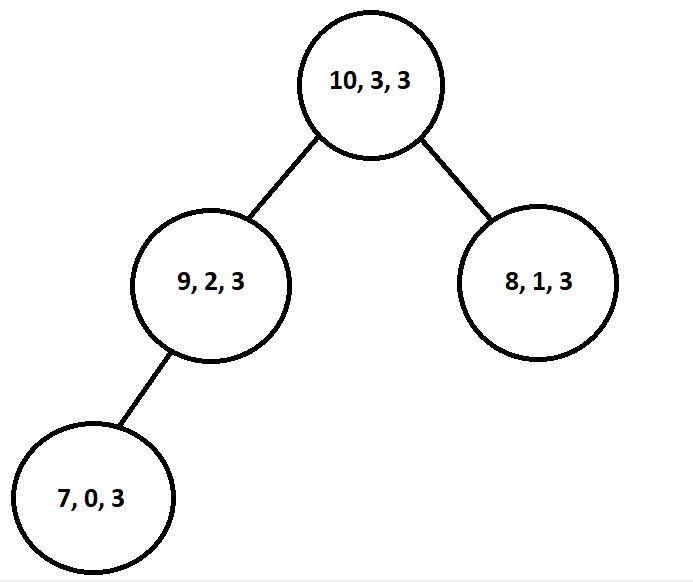# N Max Pair Combinations

## Problem Description

In this problem, we have two integer arrays/integer vectors, and our task here is to return an integer vector of a size similar to the arrays and containing the max pair sum elements of the two arrays.

We’ll take one element from vector 1 and another element from vector 2 in such a way that the sum of both elements is maximum and then put the sum in the result vector. Next time, we’ll select another pair of elements such that their sum is the second maximum, and we’ll push it in the result vector; this will go on until there are n elements (size of vector 1 and vector 2) in the result vector.

### Example

A = [1, 4, 2, 3]

B = [2, 5, 1, 6]

The max sum vector will be [10, 9, 9, 8] for the following two vectors.

Here, 10 is the maximum pair sum we can get by adding 4 and 6 from vectors A and B, respectively.

After that, the second max pair sum that we can get here is either by adding 4 with 5 or by adding 3 with 6, so we’ll add 9 two times in the resultant vector.

Finally, the fourth element of the vector will be 8, which we’ll get after adding 3 and 5 from A and B, respectively.

vec = 4 (A) + 6 (B) = 10,

vec = 3 (A) + 6 (B) = 9,

vec = 5 (A) + 4 (B) = 9, and

vec = 3 (A) + 5 (B) = 8.

## Algorithm

Heap or the Priority queue is the first thing that comes to mind when asked to find some maximum or minimum element efficiently in O(1) time. Hence, since we have to find N maximum pair sum, we’ll formulate an approach using a max heap.

The first step we’ll be to sort the given two vectors because since we are trying to find the maximum sum, sorting is an excellent idea to start.

Next, we’ll initialize a max priority queue (max heap). We’ll use this priority queue to store a tuple containing three elements (sum of the pair, element index in vector A, element index in vector B).

Now, we’ll populate the priority queue with the elements of vector A added with the maximum element in vector B. For instance, if we vector A = {1, 4, 2, 3} and vector B = {2, 5, 1, 6} then the max heap will look like this-In the next step, we’ll declare a result vector and push the top of the priority queue (maximum sum) in the vector; after pushing, we’ll also remove this from the priority queue. We’ll iterate the above step n times.

Each of the new tuples contains the sum of the maximum element in vector A and the next maximum element of vector B (decreasing index means we take one element smaller than the maximum element each time).

Finally, we return the result vector!

Sample Input 1

Sample Output 1

Sample Input 2

Sample Output 2

## Complexity Analysis

### Time Complexity

The time complexity of the above approach will be O(nlogn) since we are sorting the vectors. But if we get sorted arrays, then the time complexity will be O(n).

### Space Complexity

The space complexity of this approach to return the maximum pair sum vector is O(n) because we are using extra space here.

How do you define a max heap?

In simple terms, a max heap is a complete binary tree in which the value of each node is greater than or equal to the value of both of its children. The root node of a max heap contains the maximum element. We implement the maximum priority queues using max heaps.

What is the time complexity of finding the maximum element from a priority queue?

The time complexity of getting the maximum element from a priority queue is O(1); because of this constant time property, it is the most preferred data structure in the event of finding the maximum or the minimum from a set of elements.

What is the difference between a Min Heap and a Max Heap?

The only difference between a Min Heap and a Max Heap is the heap-ordering property. In the case of a min-heap, the minimum element is always at the top of the tree. The value of each node is less than the value of both of its children, whereas, in the case of a max heap, the value of every node is greater than its children, and the maximum element is at the top of the tree.

What is the Heap order property?

Heap order property states that the value of every node in the tree must be greater than or lesser than (depending on the type of the heap) the value of both of its children.

What are the necessary conditions for a Binary Tree to be called a Heap?

If a Binary Tree satisfies these two conditions, then it is a Heap:

1. The binary tree should be a Complete Binary Tree.
2. The binary tree should have the heap-order property.

## Key Takeaways

Today you learned one significant problem in arrays. Pat yourself on the back!! :)

This blog post discussed the N Max Pair Sum problem and an efficient approach to solve it.

A slight modification to this problem would be that you could be asked to find K pairs with maximum sum from both the arrays (where K<N) instead of N pairs. In this case, you just have to change the while condition from n-->0 to k-->0.

Do check out these informative articles on heaps too.

Converting a BST to Min Heap

Tournament Trees and Binary Heaps

If you are preparing for the upcoming Campus Placements, then don’t worry. Coding Ninjas has your back. Visit this link for a carefully crafted and designed course on-campus placements and interview preparation.